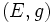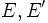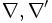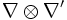# Difference between revisions of "Tensor product of metric connections is metric"

A differential manifold$M$. Two metric bundles$(E,g)$ and$(E',g')$ (i.e.,$E,E'$ are vector bundles and$g,g'$ are Riemannian metrics or pseudo-Riemannian metrics on these).$\nabla, \nabla'$ are metric connections on$(E,g)$ and$(E',g')$ respectively. Then, the tensor product of connections$\nabla \otimes \nabla'$ is a metric connection on$(E \otimes E',g \otimes g')$.